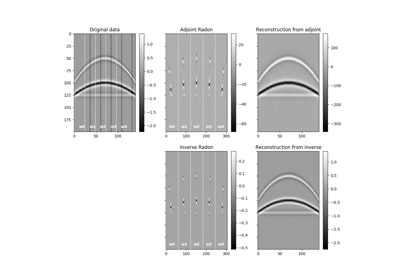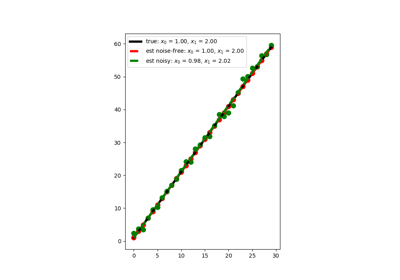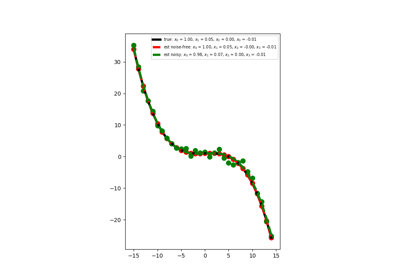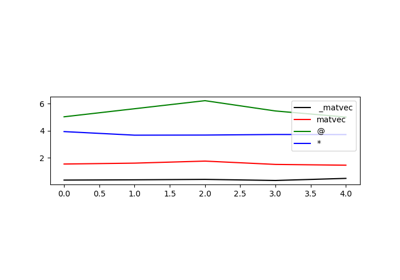# pylops.LinearOperator¶

class pylops.LinearOperator(Op=None, explicit=False)[source]

Common interface for performing matrix-vector products.

This class is an overload of the scipy.sparse.linalg.LinearOperator class. It adds functionalities by overloading standard operators such as __truediv__ as well as creating convenience methods such as eigs, cond, and conj.

Note

End users of PyLops should not use this class directly but simply use operators that are already implemented. This class is meant for developers and it has to be used as the parent class of any new operator developed within PyLops. Find more details regarding implementation of new operators at Implementing new operators.

Parameters: Op : scipy.sparse.linalg.LinearOperator or scipy.sparse.linalg._ProductLinearOperator or scipy.sparse.linalg._SumLinearOperator Operator explicit : bool Operator contains a matrix that can be solved explicitly (True) or not (False)

Methods

 __init__(self[, Op, explicit]) Initialize this LinearOperator. adjoint(self) Hermitian adjoint. cond(self, \*\*kwargs_eig) Condition number of linear operator. conj(self) Complex conjugate operator div(self, y[, niter]) Solve the linear problem $$\mathbf{y}=\mathbf{A}\mathbf{x}$$. dot(self, x) Matrix-matrix or matrix-vector multiplication. eigs(self[, neigs, symmetric, niter]) Most significant eigenvalues of linear operator. matmat(self, X) Matrix-matrix multiplication. matvec(self, x) Matrix-vector multiplication. rmatvec(self, x) Adjoint matrix-vector multiplication. transpose(self) Transpose this linear operator.
div(self, y, niter=100)[source]

Solve the linear problem $$\mathbf{y}=\mathbf{A}\mathbf{x}$$.

Overloading of operator / to improve expressivity of Pylops when solving inverse problems.

Parameters: y : np.ndarray Data niter : int, optional Number of iterations (to be used only when explicit=False) xest : np.ndarray Estimated model
eigs(self, neigs=None, symmetric=False, niter=None, **kwargs_eig)[source]

Most significant eigenvalues of linear operator.

Return an estimate of the most significant eigenvalues of the linear operator. If the operator has rectangular shape (shape!=shape), eigenvalues are first computed for the square operator $$\mathbf{A^H}\mathbf{A}$$ and the square-root values are returned.

Parameters: neigs : int Number of eigenvalues to compute (if None, return all). Note that for explicit=False, only $$N-1$$ eigenvalues can be computed where $$N$$ is the size of the operator in the model space symmetric : bool, optional Operator is symmetric (True) or not (False). User should set this parameter to True only when it is guaranteed that the operator is real-symmetric or complex-hermitian matrices niter : int, optional Number of iterations for eigenvalue estimation **kwargs_eig Arbitrary keyword arguments for scipy.sparse.linalg.eigs or scipy.sparse.linalg.eigsh eigenvalues : numpy.ndarray Operator eigenvalues.

Notes

Eigenvalues are estimated using scipy.sparse.linalg.eigs (explicit=True) or scipy.sparse.linalg.eigsh (explicit=False).

This is a port of ARPACK , a Fortran package which provides routines for quickly finding eigenvalues/eigenvectors of a matrix. As ARPACK requires only left-multiplication by the matrix in question, eigenvalues/eigenvectors can also be estimated for linear operators when the dense matrix is not available.

cond(self, **kwargs_eig)[source]

Condition number of linear operator.

Return an estimate of the condition number of the linear operator as the ratio of the largest and lowest estimated eigenvalues.

Parameters: **kwargs_eig Arbitrary keyword arguments for scipy.sparse.linalg.eigs or scipy.sparse.linalg.eigsh eigenvalues : numpy.ndarray Operator eigenvalues.

Notes

The condition number of a matrix (or linear operator) can be estimated as the ratio of the largest and lowest estimated eigenvalues:

$k= \frac{\lambda_{max}}{\lambda_{min}}$

The condition number provides an indication of the rate at which the solution of the inversion of the linear operator $$A$$ will change with respect to a change in the data $$y$$.

Thus, if the condition number is large, even a small error in $$y$$ may cause a large error in $$x$$. On the other hand, if the condition number is small then the error in $$x$$ is not much bigger than the error in $$y$$. A problem with a low condition number is said to be well-conditioned, while a problem with a high condition number is said to be ill-conditioned.

conj(self)[source]

Complex conjugate operator

Returns: eigenvalues : pylops.LinearOperator Complex conjugate operator

## Examples using pylops.LinearOperator¶2D and 3D SlidingLinear RegressionPolynomial Regression01. The LinearOpeator